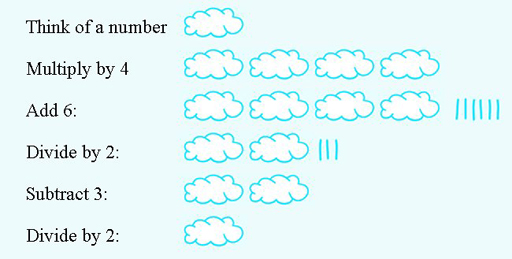Succeed with maths: part 2

Start this free course now. Just create an account and sign in. Enrol and complete the course for a free statement of participation or digital badge if available.

# 2.2 Another number trick

Here is another number trick which uses the same techniques you learned in the last section to see how it works.

## Activity _unit5.2.3 Activity 6 Another number trick

Timing: Activity 6 Another number trick

Try the following trick several times; make a note of the number you started with and your final answer each time:

• Think of a number between 1 and 10 (this will work with numbers greater than 10, but the restriction is to keep the arithmetic manageable).
• Multiply by 4.
• Divide by 2.
• Subtract 3.
• Divide by 2.

What do you notice about the answer? See if you can explain why this happens, either by using a diagram or by writing down the expressions for the answer at each stage, replacing your initial number with n again.

You should find that this time the answer is always the number you chose at the start. Both ways of showing why the number trick works are shown below.Figure _unit5.2.2 Figure 6 Graphic representation of how the number trick works
Table _unit5.2.3 Table 2 Table format showing how the number trick works
Instruction Expression
Think of a number between 1 and 10
Multiply by 4
Divide by 2

Subtract 3
Divide by 2

These two number tricks are sets of instructions that can be used to build, or derive, a formula. They are in essence not much different from those you looked at for spreadsheets. They just involved a few more steps to reach the answer.

Sometimes the answer and the set of instructions are available, while, for some reason, the starting value is not known. For example, imagine something had happened to your bank balance spreadsheet and the starting balance was no longer displayed but you still knew the final balance and all the formulas. Could you then rebuild the spreadsheet to work out initial balance? The next section shows you how.